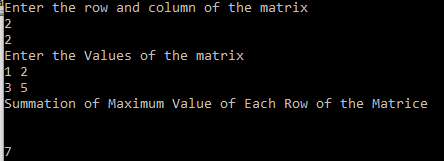# How to Calculate Summation of Maximum Value of Each Row of a Matrice in C LanguageHi guys , I just tried to code How to Calculate Summation of Maximum Value of Each Row of a Matrice in C Language .
Check the screenshot I’ve attached above .

I hope you like it , and just let me know in comment if you have any better solution .

#include <stdio.h>

int main ()
{
int array;
int i, j, m, n, sum = 0,sum2=0 ,max=0 , max2=0;

printf(“Enter the row and column of the matrix\n”);
scanf(“%d %d”, &m, &n);
printf(“Enter the Values of the matrix\n”);
for (i = 0; i < m; i++)
{
for (j = 0; j < n; j++)
{
scanf(“%d”, &array[i][j]);
}
}

for (i=0;i<m;i++)
{
for (j = 0; j < n; j++)
{
if (array[i][j] > max)
{
max=array[i][j];
}
}

max2=max2+max;
max=0;

}
printf(“Summation of Maximum Value of Each Row of the Matrice  \n”);
printf(“\n \n%d”,max2);

}